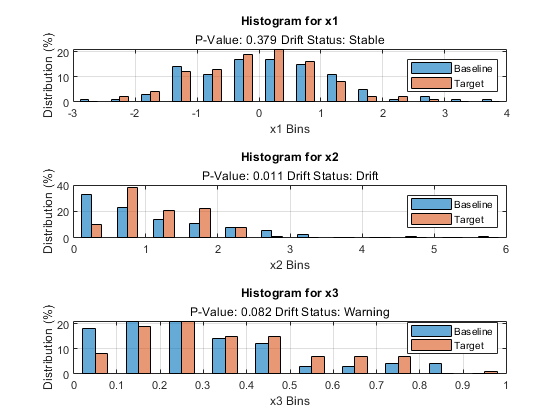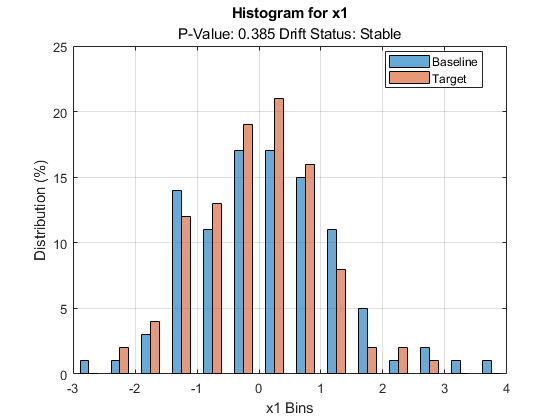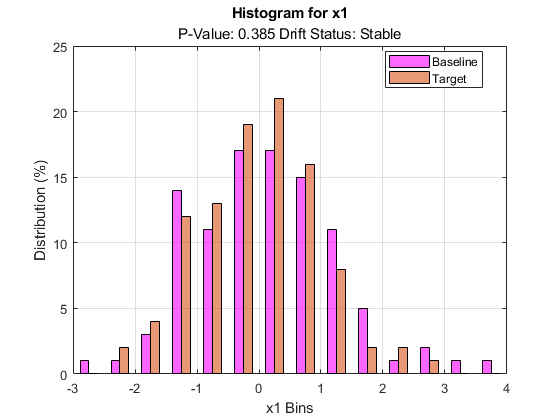# plotHistogram

Visualize histogram for a variable in drift detection

## Syntax

``plotHistogram(DDiagnostics)``
``plotHistogram(DDiagnostics,Variable=variable)``
``plotHistogram(ax,___)``
``H = plotHistogram(___)``

## Description

example

````plotHistogram(DDiagnostics)` plots a histogram of the baseline and target data for the variable with the lowest p-value.If you set the value of `'EstimatePValues'` to `false` in the call to `detectdrift`, then `plotHistogram` displays `NaN` for the p-value and the drift status.```
````plotHistogram(DDiagnostics,Variable=variable)` plots the histogram of the baseline and target data for the variable specified by `variable`.```

example

````plotHistogram(ax,___)` plots into axes `ax` instead of `gca`.```

example

````H = plotHistogram(___)` returns an array of `Histogram` objects in `H`. Use this to inspect and adjust the properties of the histogram. For more information on the `Histogram` object properties, see Histogram Properties.```

## Examples

collapse all

Generate baseline and target data with three variables, where the distribution parameters of the second and third variables change for target data.

```rng('default') % For reproducibility baseline = [normrnd(0,1,100,1),wblrnd(1.1,1,100,1),betarnd(1,2,100,1)]; target = [normrnd(0,1,100,1),wblrnd(1.2,2,100,1),betarnd(1.7,2.8,100,1)];```

Perform permutation testing for all variables to check for any drift between the baseline and target data.

`DDiagnostics = detectdrift(baseline,target)`
```DDiagnostics = DriftDiagnostics VariableNames: ["x1" "x2" "x3"] CategoricalVariables: [] DriftStatus: ["Stable" "Drift" "Warning"] PValues: [0.3850 0.0050 0.0910] ConfidenceIntervals: [2x3 double] MultipleTestDriftStatus: "Drift" DriftThreshold: 0.0500 WarningThreshold: 0.1000 Properties, Methods ```

Plot the histogram for the default variable.

`plotHistogram(DDiagnostics)``plotHistogram` by default plots the histogram of the baseline and target data for the variable with the lowest p-value. It also displays the p-value and the drift status for that variable.

Generate baseline and target data with three variables, where the distribution parameters of the second and third variables change for target data.

```rng('default') % For reproducibility baseline = [normrnd(0,1,100,1),wblrnd(1.1,1,100,1),betarnd(1,2,100,1)]; target = [normrnd(0,1,100,1),wblrnd(1.2,2,100,1),betarnd(1.7,2.8,100,1)];```

Perform permutation testing for all variables to check for any drift between the baseline and target data. Use the Energy statistic as the metric.

`DDiagnostics = detectdrift(baseline,target,"ContinuousMetric","energy")`
```DDiagnostics = DriftDiagnostics VariableNames: ["x1" "x2" "x3"] CategoricalVariables: [] DriftStatus: ["Stable" "Drift" "Warning"] PValues: [0.3790 0.0110 0.0820] ConfidenceIntervals: [2x3 double] MultipleTestDriftStatus: "Drift" DriftThreshold: 0.0500 WarningThreshold: 0.1000 Properties, Methods ```

Plot the histograms for all three variables in a tiled layout.

```tiledlayout(3,1); ax1 = nexttile; plotHistogram(DDiagnostics,ax1,Variable="x1") ax2 = nexttile; plotHistogram(DDiagnostics,ax2,Variable="x2") ax3 = nexttile; plotHistogram(DDiagnostics,ax3,Variable="x3")```Generate baseline and target data with three variables, where the distribution parameters of the second and third variables change for target data.

```rng('default') % For reproducibility baseline = [normrnd(0,1,100,1),wblrnd(1.1,1,100,1),betarnd(1,2,100,1)]; target = [normrnd(0,1,100,1),wblrnd(1.2,2,100,1),betarnd(1.7,2.8,100,1)];```

Perform permutation testing for all variables to check for any drift between the baseline and target data.

`DDiagnostics = detectdrift(baseline,target)`
```DDiagnostics = DriftDiagnostics VariableNames: ["x1" "x2" "x3"] CategoricalVariables: [] DriftStatus: ["Stable" "Drift" "Warning"] PValues: [0.3850 0.0050 0.0910] ConfidenceIntervals: [2x3 double] MultipleTestDriftStatus: "Drift" DriftThreshold: 0.0500 WarningThreshold: 0.1000 Properties, Methods ```

Plot histogram for the first variable.

`H = plotHistogram(DDiagnostics,Variable=1)````H = 2x1 Bar array: Bar (Baseline) Bar (Target) ```

You can access `H` in the workspace by double clicking on it and change `Bar` object properties. You can also make changes programmatically. For example, change the color of the histogram bars for baseline data.

`H(1).FaceColor = [1 0 1];`## Input Arguments

collapse all

Diagnostics of the permutation testing for drift detection, specified as a `DriftDiagnostics` object returned by `detectdrift`.

Variable for which to plot the histogram, specified as a string, a character vector, or an integer index.

Example: `Variable="x2"`

Example: `Variable=2`

Data Types: `single` | `double` | `char` | `string`

Axes for `plotHistogram` to plot into, specified as an `Axes` or `UIAxes` object. If you do not specify `ax`, then `plotHistogram` creates the plot using the current axes. For more information on creating an axes object, see `axes` and `uiaxes`.

## Algorithms

• For categorical data, `detectdrift` adds 0.5 correction factor to histogram bin counts for each bin to handle empty bins (categories). This is equivalent to the assumption that the parameter p, probability that value of the variable would be in that category, has the prior distribution Beta(0.5,0.5), i.e. Jefferys prior assumption for the distribution parameter.

• `plotHistogram` treats a variable as ordinal for visualization purposes under any of the following cases:

• If the variable is ordinal in either baseline or target data and the categories from baseline and target data are the same.

• If the variable is ordinal in either in baseline or target data and the categories of the other data set is a subset of the ordinal data.

• If the variable is ordinal in both baseline and target data and categories from either one is a subset of the other.

• If a variable is ordinal, `plotHistogram` preserves the order of the bin names.

## Version History

Introduced in R2022a